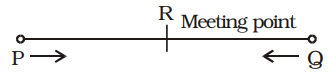## Speed, Time and Distance

#### Speed, Time and Distance

1. Two places P and Q are 162 km apart. A train leaves P for Q and simultaneously another train leaves Q for P. They meet at the end of 6 hours. If the former train travels 8 km/hour faster than the other, then speed of train from Q is
1.  12 5 km/hour 6
2.  10 5 km/hour 6
3.  9 1 km/hour 2
4.  8 1 km/hour 2

1.Speed of train starting from Q = x kmph
∴  Speed of train starting from P = (x + 8) kmph
According to the question,
PR + RQ = PQ
⇒  (x + 8) × 6 + x × 6 = 162
[Distance = Speed × Time]
⇒  6x + 48 + 6x = 162
⇒  12x = 162 – 48 = 114

 ⇒  x = 114 = 19 12 2

 = 9 1 kmph 2

##### Correct Option: CSpeed of train starting from Q = x kmph
∴  Speed of train starting from P = (x + 8) kmph
According to the question,
PR + RQ = PQ
⇒  (x + 8) × 6 + x × 6 = 162
[Distance = Speed × Time]
⇒  6x + 48 + 6x = 162
⇒  12x = 162 – 48 = 114

 ⇒  x = 114 = 19 12 2

 = 9 1 kmph 2

1. P and Q starting simultaneously from two different places proceed towards each other at a speed of 20 km/hour and 30 km/hour respectively. By the time they meet each other. Q has covered 36 km more than that of P. The distance (in km.) between the two places is
1. 144
2. 162
3. 180
4. 108

1. Let P and Q meet after t hours.
Distance = speed × time
According to the question,
30t – 20t = 36
⇒  10t = 36

 ⇒  t = 36 = 3.6 hours 10

∴  Distance between P and Q
= 30t + 20t
= 50t = (50 × 3.6) km.
= 180 km.

Second Method :
Here, a = 30, b = 20, d = 36
 Required distance =a + b× d a − b

 =30 + 20× 36 30 − 20

 = 50 × 36 = 180 km 10

##### Correct Option: C

Let P and Q meet after t hours.
Distance = speed × time
According to the question,
30t – 20t = 36
⇒  10t = 36

 ⇒  t = 36 = 3.6 hours 10

∴  Distance between P and Q
= 30t + 20t
= 50t = (50 × 3.6) km.
= 180 km.

Second Method :
Here, a = 30, b = 20, d = 36
 Required distance =a + b× d a − b

 =30 + 20× 36 30 − 20

 = 50 × 36 = 180 km 10

1. Two trains X and Y start from Jodhpur to Jaipur and from Jaipur to Jodhpur respectively. After passing each other they take 4 hours 48 minutes and 3 hours 20 minutes to reach Jaipur and Jodhpur respectively. If X is moving at 45 km/hr, the speed of Y is
1. 60 km/hr
2. 58 km/hr
3. 54 km/hr
4. 64.8 km/hr

1.  Speed of X Speed of Y

 = √ Time taken by Y Time taken by X

 ⇒ 45 = √ 3 hours 20 min. y 4 hours 48 min.

 ⇒ 45 = √ 200 minutes y 288 minutes

 = 10 12

⇒  10y = 12 × 45
 ⇒  y = 12 × 45 = 54 kmph 10

##### Correct Option: C

 Speed of X Speed of Y

 = √ Time taken by Y Time taken by X

 ⇒ 45 = √ 3 hours 20 min. y 4 hours 48 min.

 ⇒ 45 = √ 200 minutes y 288 minutes

 = 10 12

⇒  10y = 12 × 45
 ⇒  y = 12 × 45 = 54 kmph 10

1. A train running at the speed of 84 km/hr passes a man walking in opposite direction at the
speed of 6 km/hr in 4 seconds. What is the length of train (in metre) ?
1. 150
2. 120
3. 100
4. 90

1. Relative speed = (84 + 6) = 90 kmph

 =90 × 5m/sec. 18

= 25 m/sec.
∴  Length of train
= Relative speed × Time
= 25 × 4 = 100 metre

##### Correct Option: C

Relative speed = (84 + 6) = 90 kmph

 =90 × 5m/sec. 18

= 25 m/sec.
∴  Length of train
= Relative speed × Time
= 25 × 4 = 100 metre

1. Two trains, each of length 125 metre, are running in parallel tracks in opposite directions. One train is running at a speed 65 km/hour and they cross each other in 6 seconds. The speed of the other train is
1. 75 km/hour
2. 85 km/hour
3. 95 km/hour
4. 105 km/hour

1. Total length of both trains = 250 metres
Let speed of second train =x kmph
Relative speed = (65 + x) kmph

 = (65 + x) × 5 m/sec 18

 ∴ Time = Sum of length of trains Relative Speed

 ⇒ 6 = 250 (65 + x) × 5 18

 ⇒  6 × 5 × (65 + x) = 250 18

 ⇒  65 + x = 250 × 3 5

⇒  65 + x = 150
⇒  x = 150 – 65 = 85 kmph

##### Correct Option: B

Total length of both trains = 250 metres
Let speed of second train =x kmph
Relative speed = (65 + x) kmph

 = (65 + x) × 5 m/sec 18

 ∴ Time = Sum of length of trains Relative Speed

 ⇒ 6 = 250 (65 + x) × 5 18

 ⇒  6 × 5 × (65 + x) = 250 18

 ⇒  65 + x = 250 × 3 5

⇒  65 + x = 150
⇒  x = 150 – 65 = 85 kmph